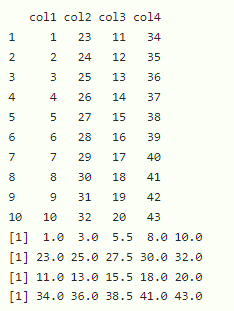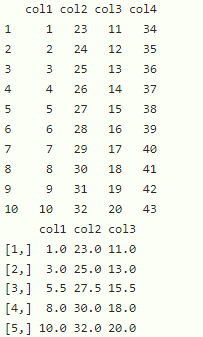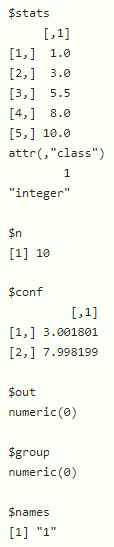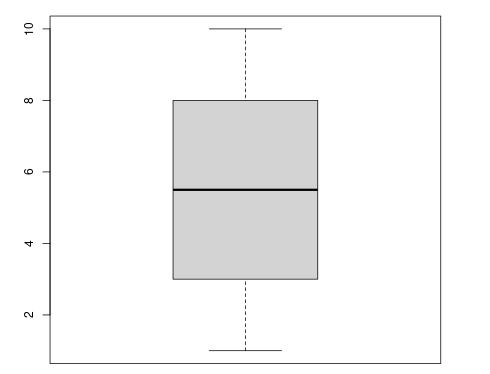GeeksforGeeks App
Open AppBrowser
Continue

# How to Calculate Five Number Summary in R?

In this article, we will discuss how to calculate five number summary in R programming language.

Five number summary is also known as a boxplot. it will return five values that are :

• The minimum value present in the given data
• The first quartile value present in the given data
• The median  value present in the given data
• The third quartile value  present in the given data
• The maximum value present in the given data

## Method 1 : Using fivenum()

This function will get the five-number summary of the given data

Syntax:

`fivenum(data)`

Example 1: Get the fivenumber summary of the vector

## R

 `# create the vector with 10 elements``data=``c``(1:10)` `# get five summary``print``(``fivenum``(data))`

Output:

`  1.0  3.0  5.5  8.0 10.0`

Example 2: Get the fivenumber summary in the dataframe

## R

 `# create the dataframe with 4 columns``data=``data.frame``(col1=``c``(1:10),col2=``c``(23:32),``                ``col3=``c``(11:20),col4=``c``(34:43))` `# display``print``(data)` `# get five summary of col1``print``(``fivenum``(data\$col1))` `# get five summary of col2``print``(``fivenum``(data\$col2))` `# get five summary of col3``print``(``fivenum``(data\$col3))` `# get five summary of col4``print``(``fivenum``(data\$col4))`

Output:We can also get fivenumber summary from multiple columns at a time by using sapply with fivenum() function

Syntax:

sapply(dataframe, fivenum)

Example: Get fivenumber summary from multiple columns at a time

## R

 `# create the dataframe with 4 columns``data=``data.frame``(col1=``c``(1:10),col2=``c``(23:32),``                ``col3=``c``(11:20),col4=``c``(34:43))` `# display``print``(data)` `# get five summary of col1,col2,col3``print``(``sapply``(data[``c``(``'col1'``,``'col2'``,``'col3'``)], fivenum))`

Output:## Method 2: Using boxplot()

This will generate a plot that represents minimum, maximum, median, first, and third quartiles

Syntax:

`boxplot(data)`

Example: Get the fivenum summary of the vector

## R

 `# create the vector with 10 elements``data=``c``(1:10)` `# get five summary``print``(``boxplot``(data))`

Output:My Personal Notes arrow_drop_up